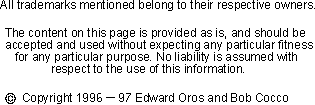Calculate Electrical Length Of A Line

The speed of a radio signal is the same as that of the speed of light. Both travel at 299,792,458 meters per second. This is a very useful number since the length of any radio signal can be determined by dividing the speed of the signal by the frequency of the signal. Throughout amateur radio though, you'll see that the numbers in this and other calculations can and often are rounded to various positions to make it easier to remember the formulas. The results are close enough to ignore the differences.
For example, let's calculate the length of a radio wave at 28.5 MHz. The formula is 300,000,000 / 28,500,000 = 10.526316 meters, or directly in MHz it would be 300 / 28.5. In order to calculate the answer in feet you could use either 984 / Frequency in MHz or a more accurate formula (the one I use) is 983.6 / Frequency in MHz. The results now are 984 / 28.5 = 34.526316 feet and 983.6 / 28.5 = 34.512281 feet. As you can see the results are very close.
Even more rounding occurs when describing which band of frequencies you are listening to. Hams are familar with bands such as 160, 80, 40, etc. and these are of course rounded numbers too. We've just seen above that when on a frequency of 28.5 you are really on the 10.526316 meter band! But of course we all call that the 10 meter band for short (thank goodness!) If you'd prefer, I suspose you could even call it the 34 feet band! No, let's not!!!
Another use of the length of a radio signal is in using transmission line sections (1/2 wave, 1/4 wave, etc.) in order to provide various impedance transformations. The quarter wave transformer is one popular such use. Now you might think that you would just take 300 / Frequency (Mhz) and divide by four to get a quarter wave line. Don't do it! You'd be off. You see, radio waves travel slower through different mediums. Which means the distance they can travel (wavelength) for a full wave is less than in free space. The coax cable you are using might for example, have a velocity factor of 66%. This indicates that the electrical length is actually 66 percent of the free space length! Be sure to figure this into your calculations.
To calculate the electrical length of the line you need to multiply the velocity factor of the line (available from the supplier of the line) by the free space length. For the length in feet, this is (983.6 / frequency in MHz) * velocity. For the length in meters, this is (299.8 / frequency in MHz) * velocity.

Switch to:

(   Speed Of Light   Divided by   Frequency    )   Times   Velocity Factory (%)

(Line Wave Length Required. Enter .25 For 1/4, .50 For 1/2, Etc.)

Your line length should be cut to

Back to the Antenna Elmer